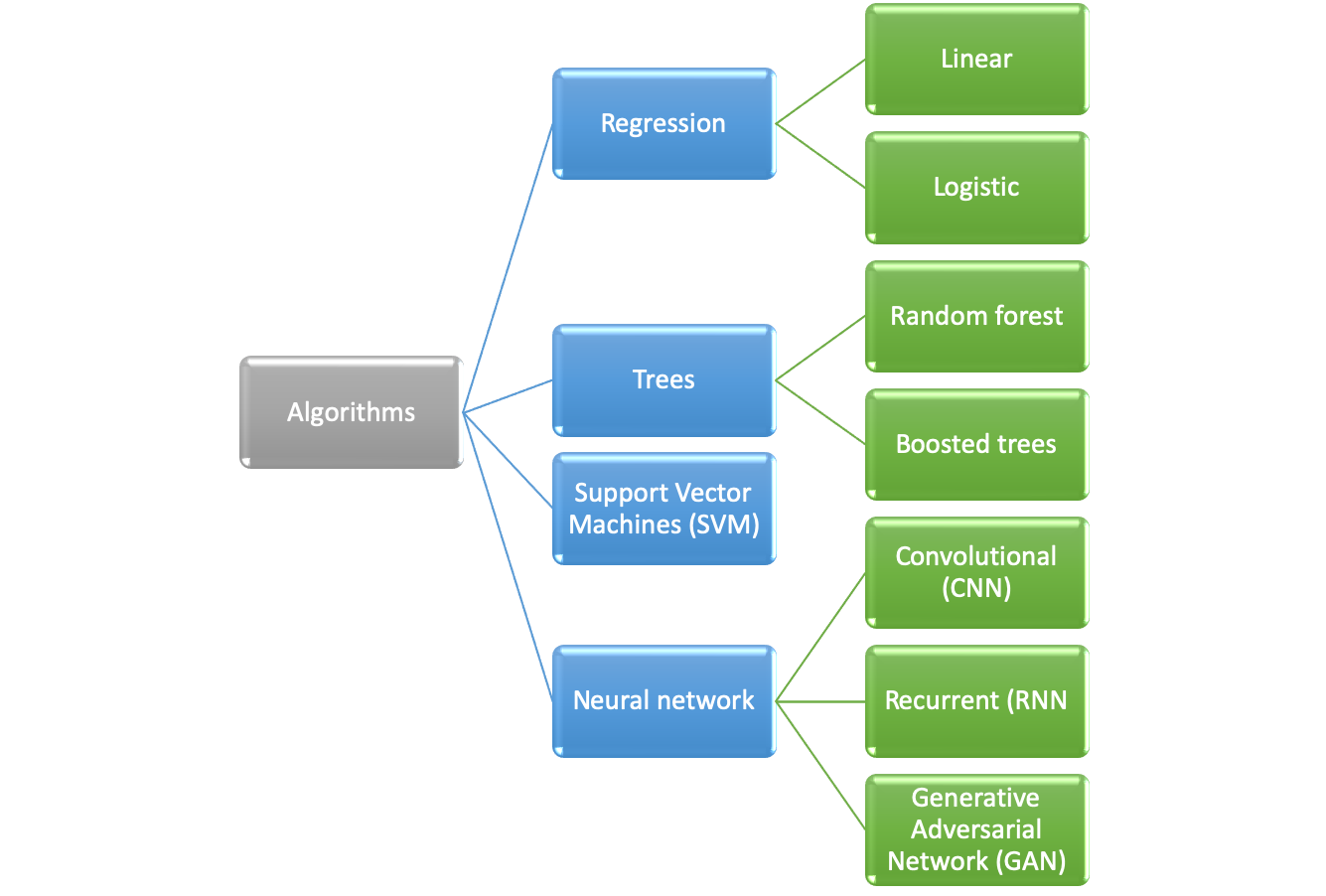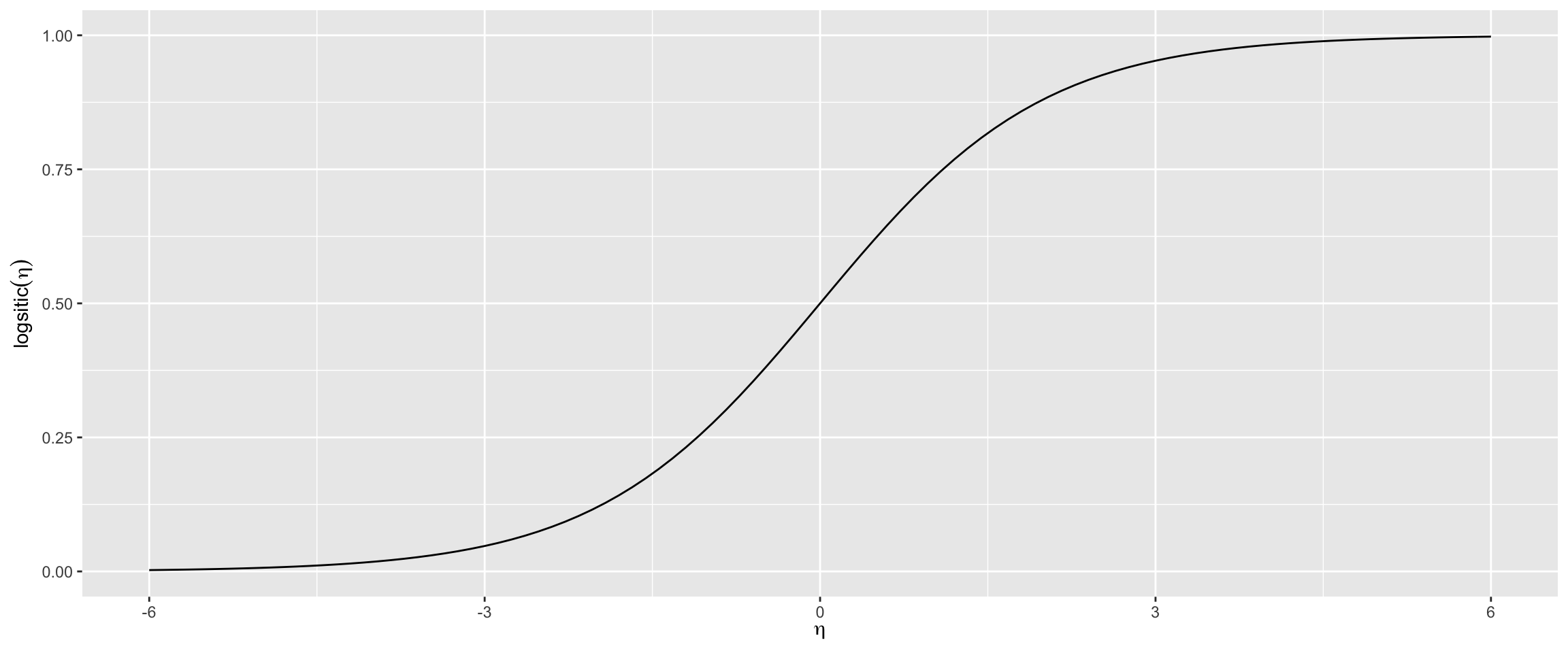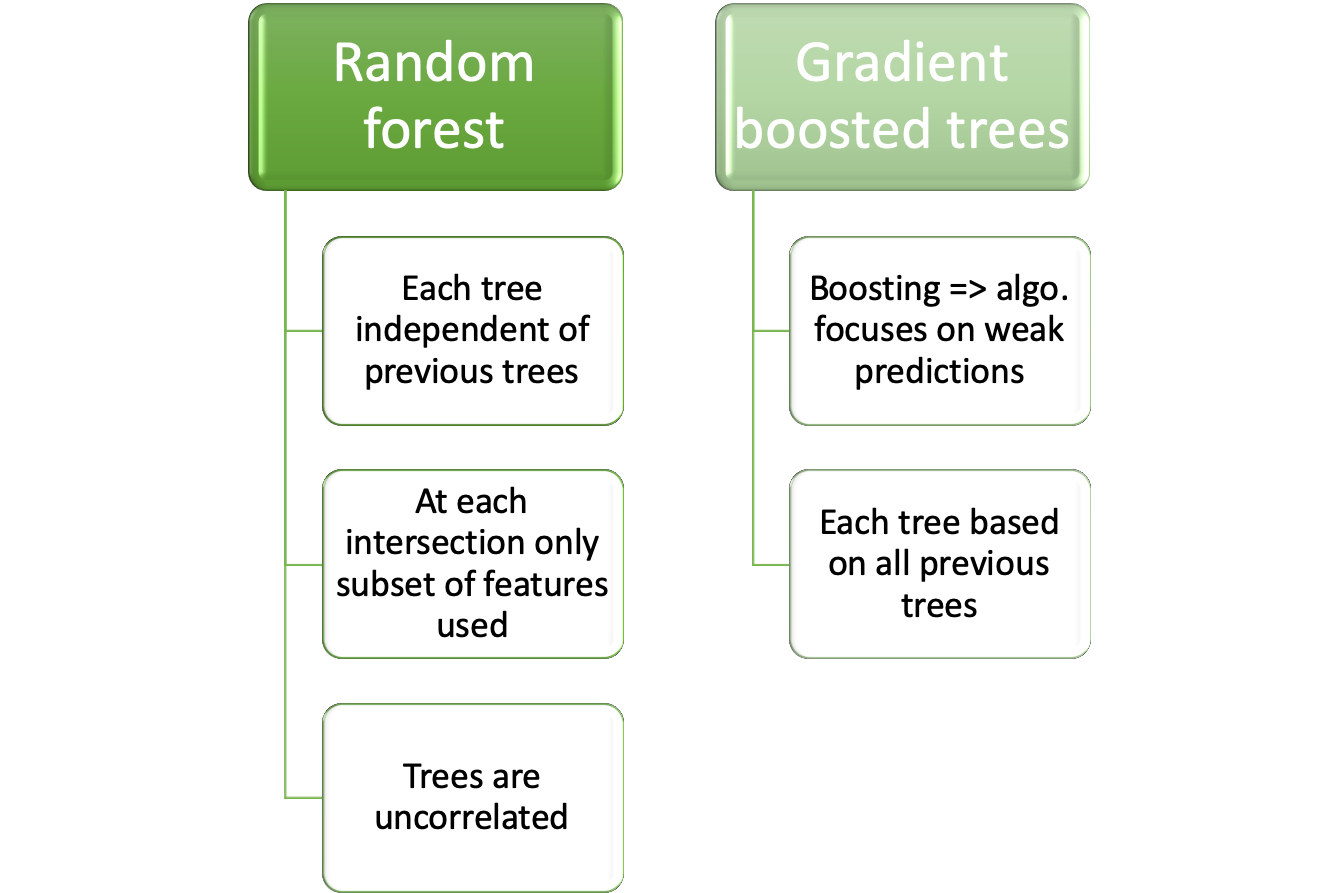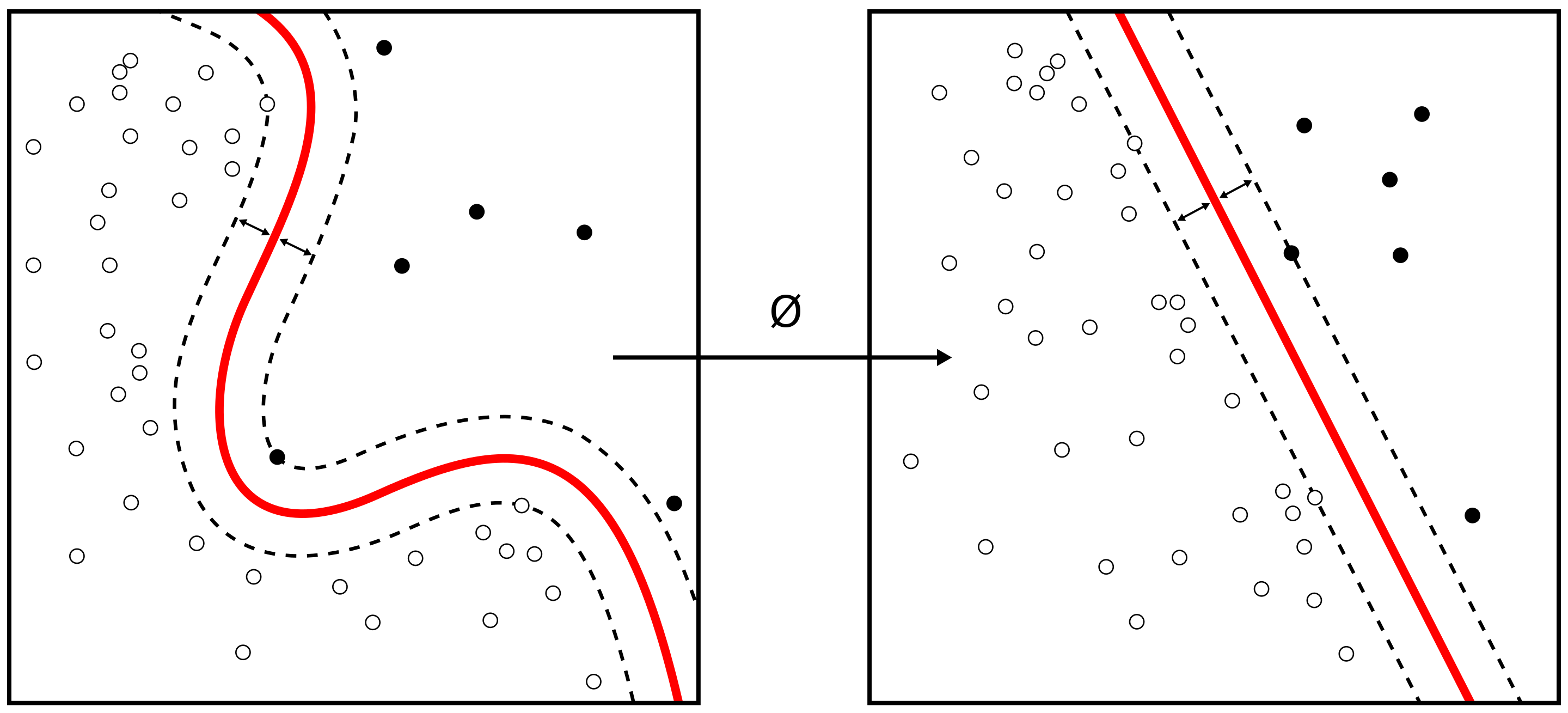## 24.3 Algorithm selection

The following algorithms were meant to be investigated following the rule to start with the least complex one.

Determination which algorithm is best suited depends on:

• doesn’t have to be a machine learning algorithm
• Use simple algorithm for feature engineering

• Use more complex algorithm if result is unsatisfactory

This is even more important since the algorithm was to be run on a satellite which where computing power is more limited than on earth### 24.3.1 Logistic regression

Logistic regression is the algorithm with the lowest computational complexity and therefore it was the algorithm with which the investigation for the suitable model would start

• Lowest computational complexity
• Start algorithm to determine suitable algorithm
• Details of algorithm are given in chapter 9.2$logistic(\eta) = \frac{1}{1+exp^{-\eta}}$

$P(Y = 1 \vert X_i = x_i) = \frac{1}{1+exp^{-(\beta_0 + \beta_1X_1+ \dots \beta_n X_n)}}$

where:

• $$\beta_n$$ are the coeffcients we are searching
• $$X_n$$ are the features

### 24.3.2 Tree based

Two dominant concepts are used for tree based algorithms:Details on the algorithms are given at:

Details on the algorithms:

• Random forest in chapter 9.3.3
• Gradient boosted trees in chapter 9.3.4

### 24.3.3 Support Vector Machine (SVM) TBD

• Support vector machine in chapter 9.4Figure from Alisneaky, svg version by User:Zirguezi [CC BY-SA (https://creativecommons.org/licenses/by-sa/4.0)]

Constrained optimization problem

$\max_{\\beta_{1}, \ldots, \beta_{p}} M$

$\textrm{subject to} \sum_{j=1}^{p} \beta_{j}^{2}=1$

$y_{i}\left(\beta_{0}+\beta_{1} x_{i 1}+\ldots+\beta_{p} x_{i p}\right) \geq M$ for all i=1, $$\ldots$$, N.